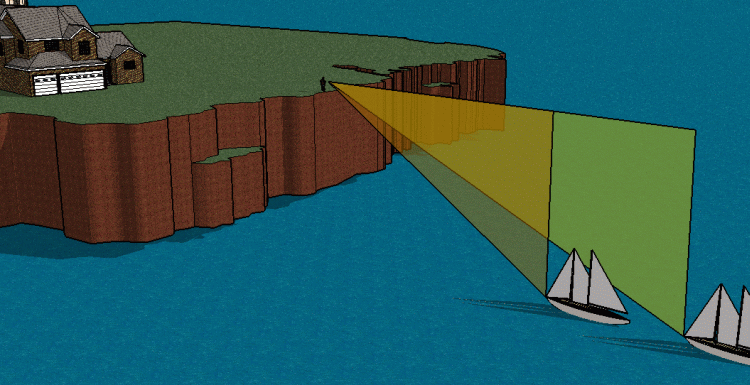SEARCH HOMEMath Central Quandaries & QueriesQuestion from joyce, a student: From a cliff 150 ft above a lake, we see a boat sailing directly towards us. The angle of depression of the boat is seen to be 5 degrees and 7 inches and 11 degrees and 18 inches. Find the distance sailed between observations. THANK YOU IN ADVANCE!!!!Hi Joyce. Here's another sketch showing you the right triangles involved:Both triangles have the same height (150 ft). The height is the side opposite the angle of depression (declination). The difference in distances is the difference in the lengths of the legs of the right triangles that are adjacent to the angle.

Therefore, you can use the tangent function of trigonometry to find the top portion of each triangle. The differences between these lengths is the distance sailed between the two observations.

Hope this helps,
Stephen La Rocque.Math Central is supported by the University of Regina and The Pacific Institute for the Mathematical Sciences.